# Square Yards Calculator

Get the square yardage of an area by entering the measurements of a space below. Optionally, enter a price per sq yd to get an estimated material cost.

Optionally enter the price per square yard
\$

## Material Estimate:

Sq Yds
Estimated Price

Cost
Learn how we calculated this below

## Using the Square Yardage Calculator

This calculator makes short work of figuring out the square yardage of any area for all your construction, landscaping, and craft projects, such as carpet, flooring, tiling, drywalling, gardening, and even sewing.

To quickly find the square yardage of a rectangular area, simply enter the length and width of your rectangle into the calculator above. Use any units, imperial or metric. To estimate costs, enter the price per sq yd. The calculator will instantly give you the necessary square yardage of the material, as well as its cost.

## How to Find Square Yards

Square yardage is a common measurement of area and is used in many fields. One square yard is equal to 9 square feet, or an area that is three feet wide by three feet long.

To calculate square yardage, you must first convert your measurements to yards. You can use our length converter for a quick conversion, or, if you already know the area in sq ft, use our square feet to square yards calculator for instant results.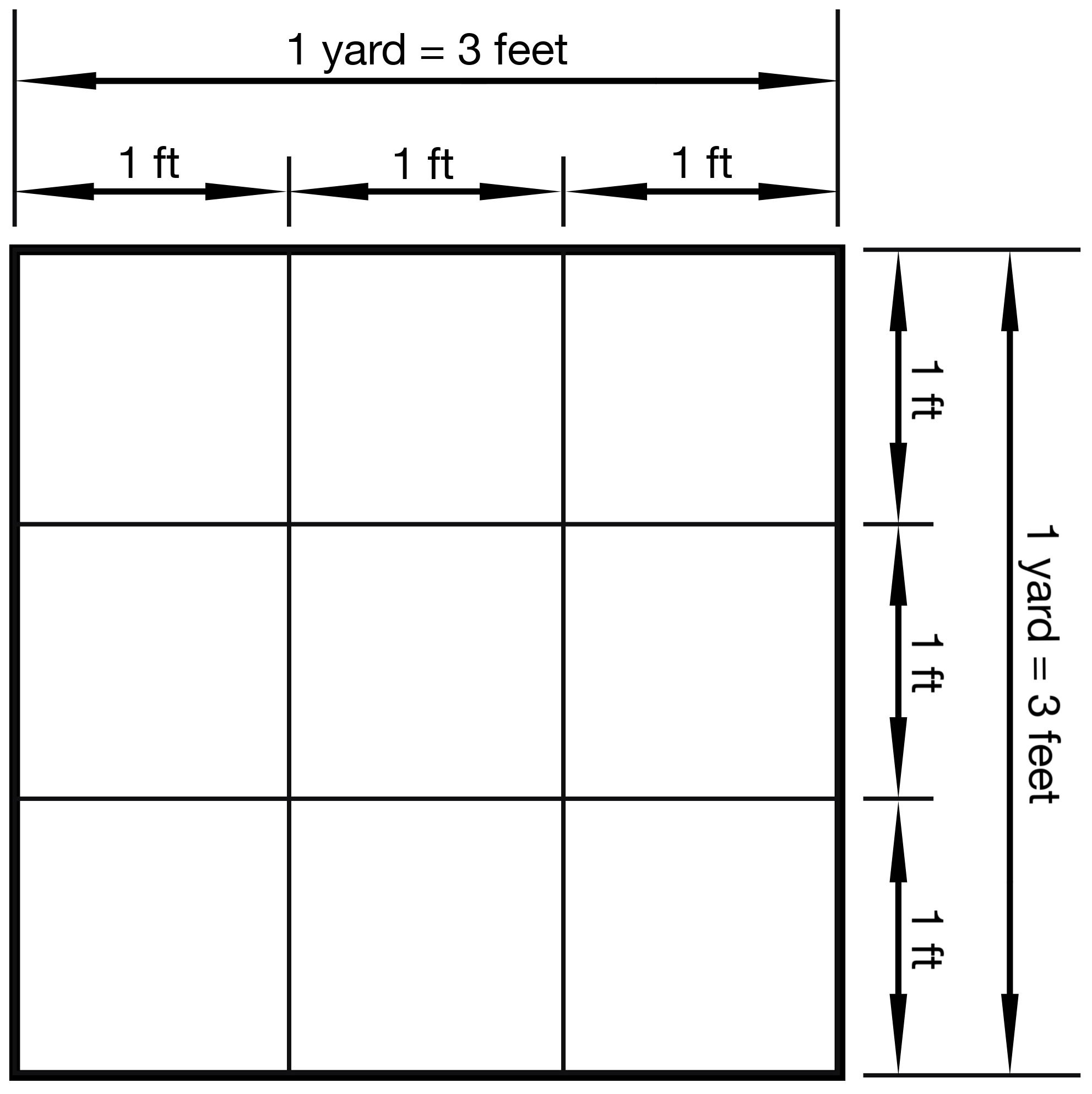Diagram demonstrating that 1 square yard is equal to a 3 ft x 3 ft square, or 9 sq ft.

Use these steps to calculate the area of a rectangular space in square yards.

• Measure the length and width of each side of the rectangle
• Convert these measurements to yards as described above.
• Multiply the length times the width to get the area measurement in sq yds.

sq yds = lengthyds × widthyds

## How to Find Square Yards Using Measurements in Feet

The steps above guide you to convert all measurements to yards before multiplying, but it’s possible to find square yards after multiplying if all of your measurements are in feet too. Follow these steps if all of your measurements are in feet:

• Multiply the length in feet times the width in feet to find the area in square feet
• Divide the area by 9 to find square yards.

sq yds = (length ft × width ft) ÷ 9

## Formulas to Find Square Yards

The following formulas show how to calculate area in square yards for various shapes. Formulas to find the area in square yards are provided for measurements given in yards or feet to simplify conversion.

### Rectangle

sq yds = lyds × wyds

sq yds = lft × wft ÷ 9

l = length
w = width

You can also use our rectangle area calculator.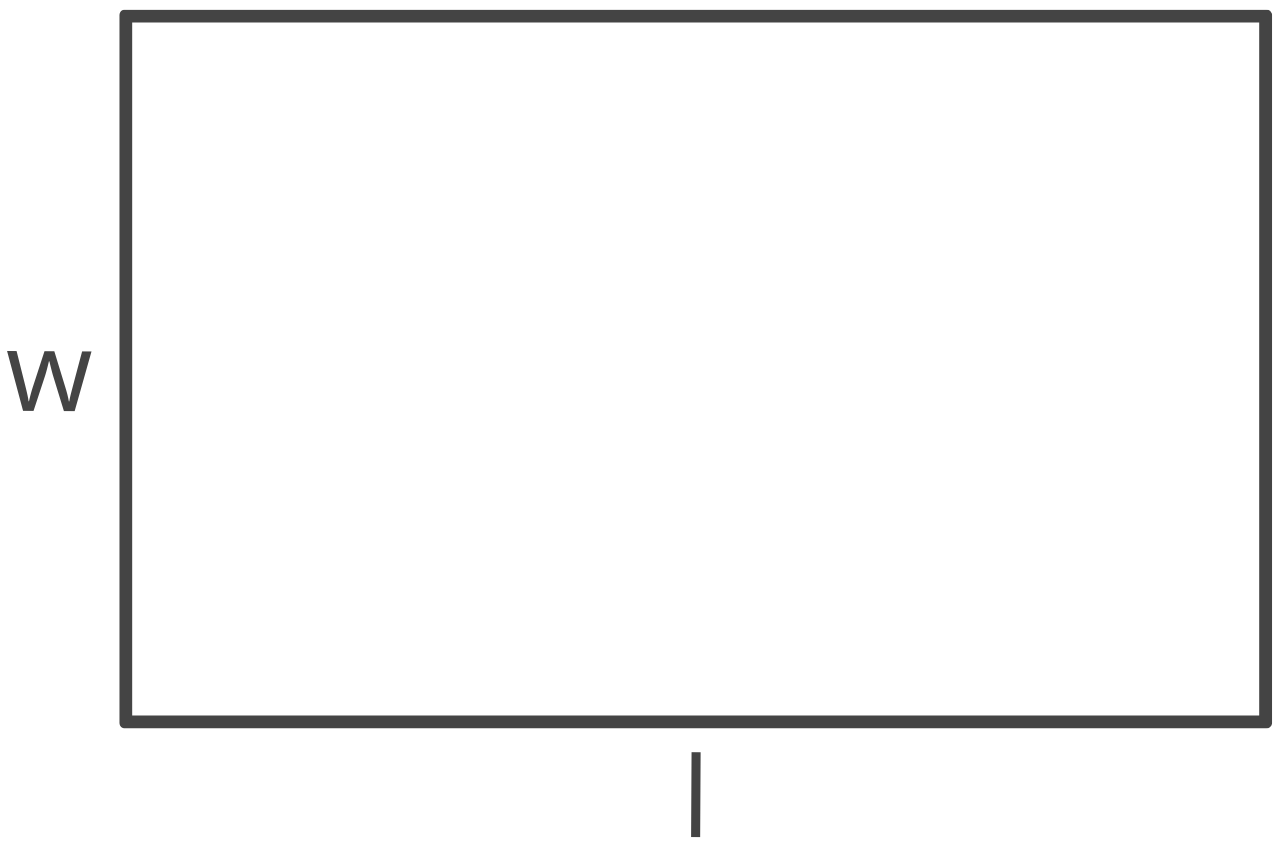### Rectangular Border

sq yds = (lyds × wyds) – (ayds × byds)

sq yds = ((lft × wft) – (aft × bft)) ÷ 9

l = outer length
w = outer width
a = inner length
b = inner width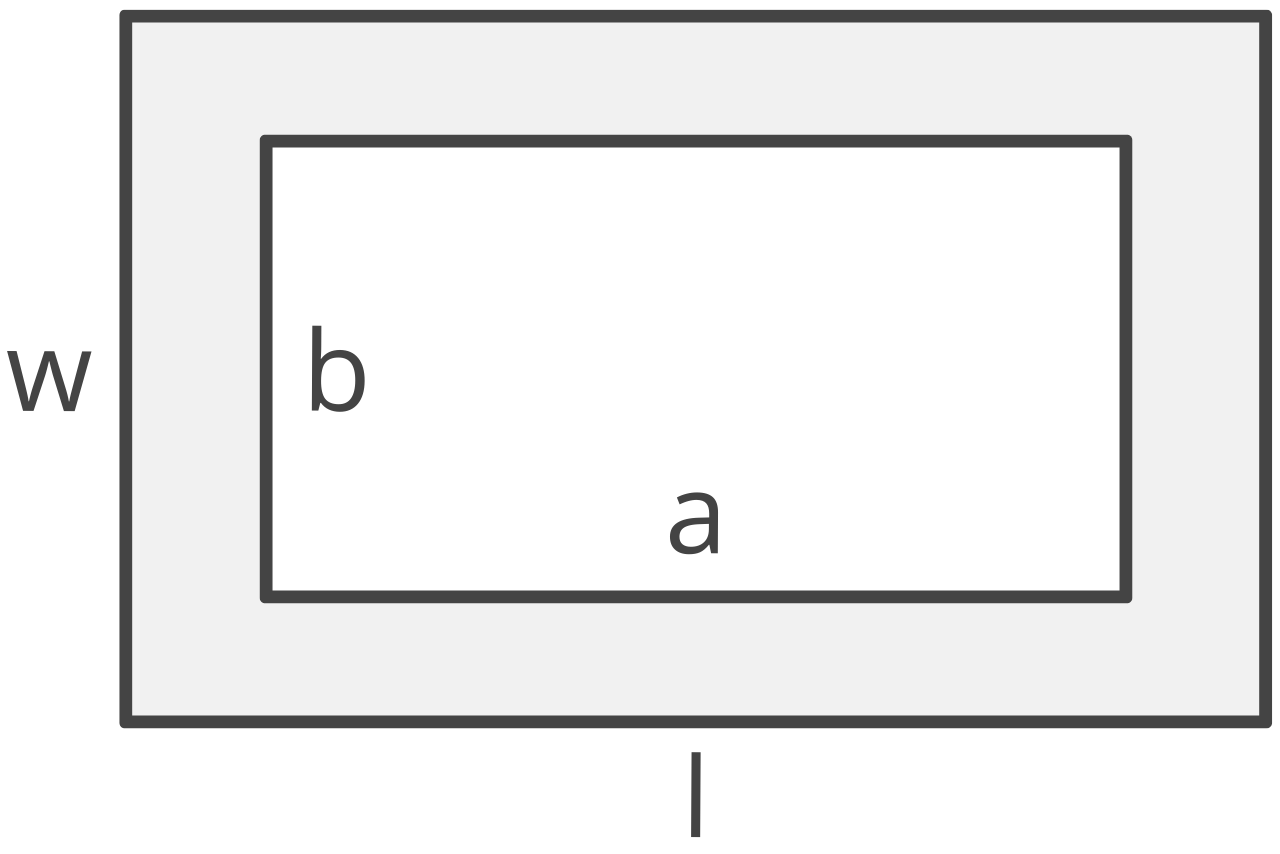### Circle

sq yds = πryds2

sq yds = πrft2 ÷ 9

π = 3.14159265359

If you know the diameter of the circle, you can find the radius by dividing the diameter in half. You can also use our circle calculator to find the radius.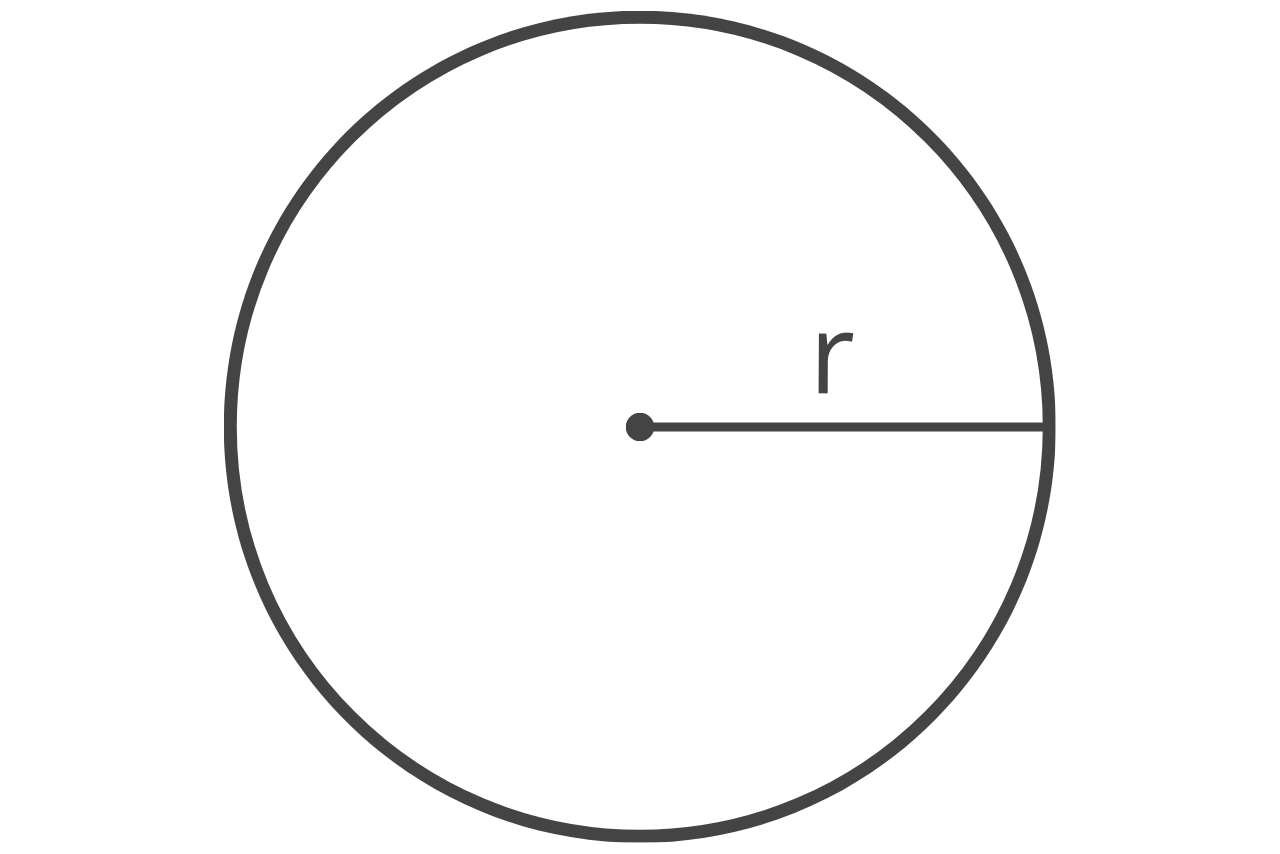### Triangle

s = 1/2(ayds + byds + cyds)
sq yds = s(s – a)(s – b)(s – c)

s = 1/2(aft + bft + cft)
sq yds = s(s – a)(s – b)(s – c) ÷ 9

a = edge a
b = edge b
c = edge c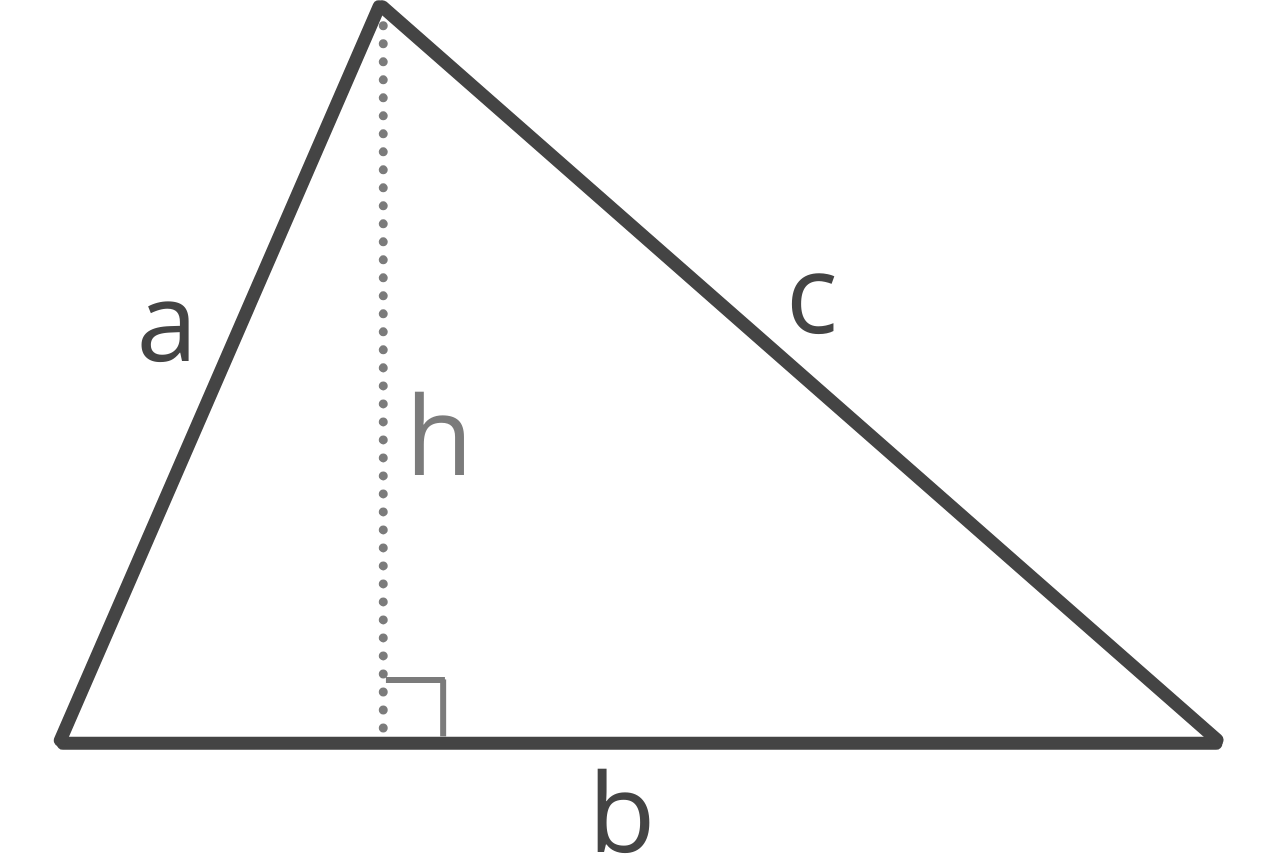Our area calculator has formulas for many more shapes.